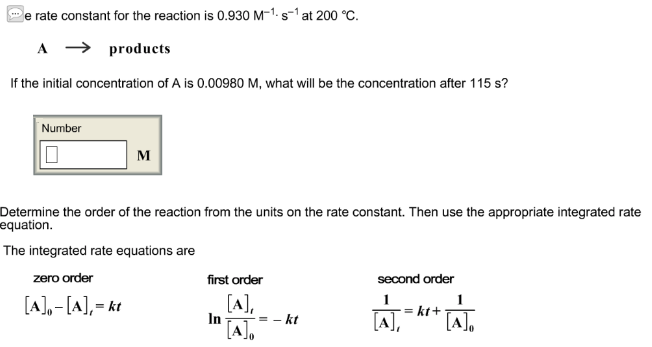# Problem: The rate constant for the reaction is 0.930 M-1• s-1 at 200°C. A → productsIf the initial concentration of A is 0.00980 M, what will be the concentration after 115 S? Determine the order of the reaction from the units on the rate constant. Then use the appropriate integrated rate equation. The integrated rate equation are

###### FREE Expert Solution
91% (299 ratings)###### Problem Details

The rate constant for the reaction is 0.930 M-1• s-1 at 200°C.

A → products

If the initial concentration of A is 0.00980 M, what will be the concentration after 115 S?

Determine the order of the reaction from the units on the rate constant. Then use the appropriate integrated rate equation.

The integrated rate equation are